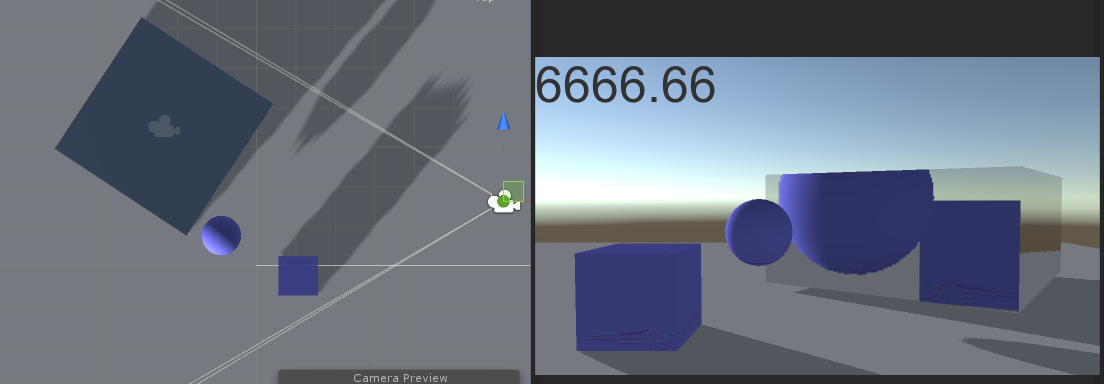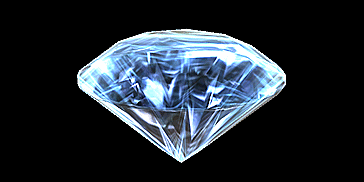# 三言两语说shader（九）钻石

1.看了stalendp blog里那篇《钻石效果》后头的参考文献

2.试验了一些简单的镜面反射3.到AssetStore搜索了下相关资源Shader "FX/Gem"
{
Properties {
_Color ("Color", Color) = (1,1,1,1)
_ReflectionStrength ("Reflection Strength", Range(0.0,2.0)) = 1.0
_EnvironmentLight ("Environment Light", Range(0.0,2.0)) = 1.0
_Emission ("Emission", Range(0.0,2.0)) = 0.0
[NoScaleOffset] _RefractTex ("Refraction Texture", Cube) = "" {}
}
SubShader {
Tags {
"Queue" = "Transparent"
}
// First pass - here we render the backfaces of the diamonds. Since those diamonds are more-or-less
// convex objects, this is effectively rendering the inside of them.
Pass {

Cull Front
ZWrite Off

CGPROGRAM
#pragma vertex vert
#pragma fragment frag
#include "UnityCG.cginc"

struct v2f {
float4 pos : SV_POSITION;
float3 uv : TEXCOORD0;
};

v2f vert (float4 v : POSITION, float3 n : NORMAL)
{
v2f o;
o.pos = mul(UNITY_MATRIX_MVP, v);

// TexGen CubeReflect:
// reflect view direction along the normal, in view space.
float3 viewDir = normalize(ObjSpaceViewDir(v));
o.uv = -reflect(viewDir, n);
o.uv = mul(_Object2World, float4(o.uv,0));
return o;
}

fixed4 _Color;
samplerCUBE _RefractTex;
half _EnvironmentLight;
half _Emission;
half4 frag (v2f i) : SV_Target
{
half3 refraction = texCUBE(_RefractTex, i.uv).rgb * _Color.rgb;
half4 reflection = UNITY_SAMPLE_TEXCUBE(unity_SpecCube0, i.uv);
reflection.rgb = DecodeHDR (reflection, unity_SpecCube0_HDR);
half3 multiplier = reflection.rgb * _EnvironmentLight + _Emission;
return half4(refraction.rgb * multiplier.rgb, 1.0f);
}
ENDCG
}

// Second pass - here we render the front faces of the diamonds.
Pass {
ZWrite On
Blend One One

CGPROGRAM
#pragma vertex vert
#pragma fragment frag
#include "UnityCG.cginc"

struct v2f {
float4 pos : SV_POSITION;
float3 uv : TEXCOORD0;
half fresnel : TEXCOORD1;
};

v2f vert (float4 v : POSITION, float3 n : NORMAL)
{
v2f o;
o.pos = mul(UNITY_MATRIX_MVP, v);

// TexGen CubeReflect:
// reflect view direction along the normal, in view space.
float3 viewDir = normalize(ObjSpaceViewDir(v));
o.uv = -reflect(viewDir, n);
o.uv = mul(_Object2World, float4(o.uv,0));
o.fresnel = 1.0 - saturate(dot(n,viewDir));
return o;
}

fixed4 _Color;
samplerCUBE _RefractTex;
half _ReflectionStrength;
half _EnvironmentLight;
half _Emission;
half4 frag (v2f i) : SV_Target
{
half3 refraction = texCUBE(_RefractTex, i.uv).rgb * _Color.rgb;
half4 reflection = UNITY_SAMPLE_TEXCUBE(unity_SpecCube0, i.uv);
reflection.rgb = DecodeHDR (reflection, unity_SpecCube0_HDR);
half3 reflection2 = reflection * _ReflectionStrength * i.fresnel;
half3 multiplier = reflection.rgb * _EnvironmentLight + _Emission;
return fixed4(reflection2 + refraction.rgb * multiplier, 1.0f);
}
ENDCG
}

// Shadow casting & depth texture support -- so that gems can
// cast shadows
UsePass "VertexLit/SHADOWCASTER"
}
}


1.折射光的模拟

2.pass设计

3.光照模型设计

4.HDR

High Dynamic Range，不负责任的说下，大概就是假如0是黑色，1是白色，那么有些部分的颜色可以超过1，这样最终画面结果会呈现出一种亮处高闪耀的效果，具体请参考Unity官方文档说明。

5.菲涅尔效果